Theory of Vibration – Ismtechbd

# Theory of Vibration

The way material vibrates under given condition is an important aspect of design of civil structures or mechanical equipment. Historically unexpected failures of bridges and columns have happened because designers did not consider effect of vibration. In this tutorial you will understand fundamentals of vibration and will learn how to apply it in mechanical / civil design you undertake.

## A Simple Experiment to Understand Nature of Vibration

Consider following spring-mass system. First spring is given a small initial stretch second spring is given more stretch. If you measure time period of oscillation for both the cases you can find it is same! Both are having a time period of 6.28 s.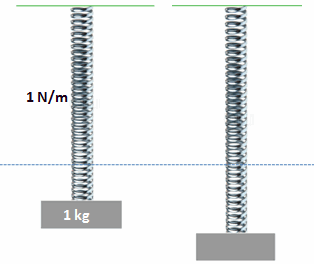Fig.1 Different initial stretch is given to the same spring-mass system and time period is measured

This is one big characteristic of vibrating systems, time period of oscillation does not depend upon initial stretch you give, it is a property of the system. More commonly used term used in vibrating world is frequency, which is defined as follows.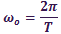Where T is time period of oscillation. Or one could say frequency of oscillation is a property of the system. More precise term is natural frequency, since the system is made up of only stiffness and inertial components. This is true even for a complicated system, say a bridge, chimney or an electronic circuit. All of them has got a property called natural frequency

## Mathematical Analysis

One can do mathematical analysis of any vibrating system with help of Newton’s 2nd law of motion. Newton’s second law motion when applied on mass lead to following differential equation.This equation has got many solutions, solution of highest significance is given below.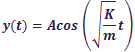Where A is initial stretch given to the spring. Graphically it is represented as shown in below.Fig.2 Graphical representation of motion of spring-mass system with time

Where Y is measured downward from equilibrium line, a line where at which gravitational force and spring force are balanced (represented by blue dotted line). Time period of oscillation and angular frequency can be derived from equation above asSo for a simple spring-mass system frequency of vibration is a function of mass and spring constant.If you substitute values of spring constant and mass of previous experiment in above equation time period of vibration can be obtained as 6.28s.

## Effect of Damping on Vibration

You can notice that vibration of mass continues forever in previous case, it never dies out. But this is not a practical case, what do you see in practice is something like this.

Amplitude of oscillation decreases with time and it finally dies out. Here we have to consider effect of air viscous force on mass in order to simulate this problem more accurately. Damping causes continuous energy loss to the system, as system loses its energy amplitude of oscillation decreases.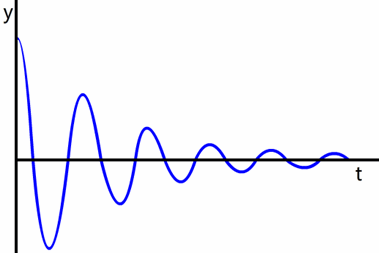Fig.3 Amplitude vibration decreases in an actual case due to effect of air viscous force

Forces acting on mass in this case shown in figure below in blue arrows. Here spring force and viscous(damping) forces are acting on the mass, damping force is proportional velocity of mass with opposite sign of velocity. Effect of air viscous force can be represented as a single viscous dash port as shown.Fig.4 Forces acting on a damped vibration case

Mathematical solution of this problem can be obtained by applying Newton’s 2nd law of motion to the system. The differential equation so obtained will beIt has got one more term compared to simple spring-mass system case, a term to incorporate viscous force on mass.

## Critical and Over Damping

Now let us use a highly viscous fluid around the mass instead of air. Here you can find that vibration dies out much faster and tendency of oscillation has decreased. As you increase viscosity of fluid further tendency for oscillation further decreases. So there will be a limiting viscosity where oscillatory behavior of mass completely disappears. This phenomenon is known as critical damping, where the mass goes back to its equilibrium position without any oscillation.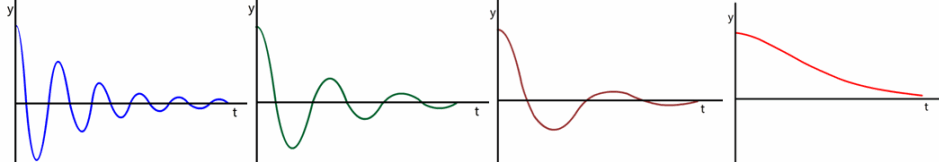Fig.5 As viscosity of surrounding fluid is increased tendency for oscillation decreases and finally a case with no oscillation is reached

Coefficient of viscosity required for achieving critical damping is given byYou can further increase viscosity of surrounding, even above critical damping limit and notice that here also mass goes to equilibrium position without any oscillation. This condition is known as overdamped.

## Effect of External Force – Resonance

When an oscillating external force is applied on mass nature of vibration will change dramatically. Here oscillating frequency will be same external force frequency, it is not related natural frequency of the system. Amplitude of the oscillation is not the initial stretch given by the user, but it will be strong function of external frequency and natural frequency. Amplitude of oscillation will become very high when external frequency and natural frequency values are very close.If external force frequency is same as natural frequency of material it will result in a phenomenon called resonance, where amplitude of oscillations become so high and it will eventually result in damage of material.

## Industrial Applications

• The Intriguing problem of Chimney Collapse
Have you ever noticed rings at top of a chimney? What does it signify?

We will go through an incident which happened almost 100 years back. A chimney which conforms to mechanical strength requirement was erected in England in 1900s. But surprising engineers who built it,the structure collapsed one day. An enquiry was launched to investigate what went wrong in design of the chimney. After a detailed research the enquiry commission came up with a startling report. Their conclusion was like this.

The collapse happened because designers did not consider effect of vibration / resonance of chimney. When wind blows across chimney during a particular wind speed range a phenomenon called Vortex shedding happens behind the chimney, here flow vortexes appear and disappear at a particular frequency. This will induce fluctuating force of same frequency on chimney. If frequency of vortex shedding is same as natural frequency of material of construction this will lead to resonance. At resonance amplitude of oscillation becomes so large that structure finally collapses. That exactly what happened for the chimney.

So what could be a solution for this danger. The obvious answer is prevent Vortex shedding phenomenon, so that the chimney will not vibrate. And the rings you see on chimney are vortex breakers, which will distract the flow and spoil vortex formation behind chimney.

1. ### Why do they spend lot of time on mass balancing?

Even if you fabricate a rotating component at high precision level there will be mass imbalance in the rotor. Centrifugal force generated by this mass imbalance is equivalent to a forced vibration case. If you do not balance this imbalance by a counter mass, it will result in vibration and damage of rotor.

## How to build a career in field of Vibration?

Excited about field of vibration? Do you want a build career in this filed ? If answers to these questions are Yes, then an exciting world of NVH (Noice,Vibration & Harshness) is awaiting you. There are 2 different career streams available according to your interest

1. #### Experimental analysis and Development

• Balancing of rotating components
• Noise testing
2. #### FEA based simulation and Development

You can simulate almost all the things you do experimentally in computers using FEA. So it is obvious that you have to have a strong knowledge in Finite Element Analysis in order to enter this job domain. Anyway before you start dreaming on this careers first thing you have to do is gain more in-depth knowledge on vibration. Hope from this website you got a good introduction to this filed. Now its time to go through some standard text books and learn more on this field.